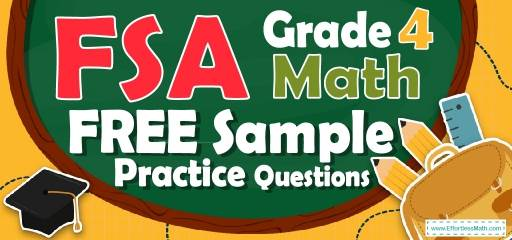# 4th Grade FSA Math FREE Sample Practice QuestionsPreparing your student for the 4th Grade FSA Math test? To succeed on the FSA Math test, students need to practice as many real FSA Math questions as possible.  There’s nothing like working on FSA Math sample questions to measure your student’s exam readiness and put him/her more at ease when taking the 4th Grade FSA Math test. The sample math questions you’ll find here are brief samples designed to give students the insights they need to be as prepared as possible for their 4th Grade FSA Math test.

Check out our sample 4th Grade FSA Math practice questions to find out what areas your student needs to practice more before taking the 4th Grade FSA Math test!

Start preparing your student for the 2022 FSA Math test with our free sample practice questions. Also, make sure to follow some of the related links at the bottom of this post to get a better idea of what kind of mathematics questions students need to practice.

## 10 Sample 4th Grade FSA Math Practice Questions

1- What is the value of A in the equation $$64 ÷ A = 8$$

A. 2

B. 4

C. 6

D. 8

2- Jason’s favorite sports team has won 0.62 of its games this season. How can Jason express this decimal as a fraction?

A. $$\frac{6}{2}$$

B.$$\frac{62}{10}$$

C. $$\frac{62}{100}$$

D. $$\frac{6.2}{10}$$

3- Use the models below to answer the question.
$$\img{https://appmanager.effortlessmath.com/public/images/questions/taar422d.JPG}$$
Which statement about the models is true?

A. Each shows the same fraction because they are the same size.

B. Each shows a different fraction because they are different shapes.

C. Each shows the same fraction because they both have 3 sections shaded.

D. Each shows a different fraction because they both have 3 shaded sections but a different number of total sections.

4- Sophia flew 2,448 miles from Los Angeles to New York City. What is the number of miles Sophia flew rounded to the nearest thousand?

A. 2,000

B. 2,400

C. 2,500

D. 3,000

5- Write $$\frac{124}{1000}$$ as a decimal number.

A. 1.24

B. 0.124

C. 12.4

D. 0.0124

6- Erik made 12 cups of juice. He drinks 3 cups of juice each day. How many days will Erik take to drink all of the juice he made?

A. 2 days

B. 4 days

C. 8 days

D. 9 days

7- Jason has prepared $$\frac{4}{10}$$ of his assignment. Which decimal represents the part of the assignment Jason has prepared?

A. 4.10

B. 4.01

C. 0.4

D. 0.04

8- Emma described a number using these clues.
• 3 digits of the number are 4, 7, and 9
• The value of the digit 4 is $$(4 × 10)$$
• The value of the digit 7 is $$(7 × 1000)$$
• The value of the digit 9 is $$(9 × 10000)$$
Which number could fit Emma’s description?

A. 9,724.04

B. 90,734.40

C. 97,040.04

D. 98,740.70

9- There are 18 boxes and each box contains 26 pencils. How many pencils are in the boxed in total?

A. 108

B. 208

C. 468

D. Not here

10- Emily and Ava were working on a group project last week. They completed $$\frac{7}{10}$$ of their project on Tuesday and the rest on Wednesday.
Ava completed $$\frac{3}{10}$$ of their project on Tuesday. What fraction of the group project did Emily complete on Tuesday?

A. $$\frac{2}{10}$$

B. $$\frac{3}{10}$$

C. $$\frac{4}{10}$$

D. $$\frac{5}{10}$$

## Best 4th Grade FSA Math Workbook Resource for 2022

1- D
$$A = 64 ÷ 8$$
$$A=8$$

2- C
0.62 is equal to $$\frac{62}{100}$$

3- D
the model for the first fraction is divided into 6 equal parts. We shade 3_6 to show the same amount as 1_2. The model for the second fraction is divided into 8 equal parts. We shade 3_ 8 that it shows these two models are different fractions.

4- A
When rounding to the nearest thousand, you will need to look at the last three digits. If the last three digits are 449 or less round to the next number that is smaller than the number given and end with three zeros.
On the other hand, If the last three digits are 500 or more, round to the next number bigger than the given number and end with three zeros.

5- B
$$\frac{124}{1000}$$ is equal to 0.124

6- B
$$12 ÷ 3 = 4$$

7- C
$$\frac{4}{10}=0.4$$

8- C
only choice C fits Emma’s description

9- C
$$18 × 26 = 468$$

10- C
$$\frac{7}{10}-\frac{3}{10}=\frac{4}{10}$$

## The Best Books to Ace the 4th Grade FSA Math Test

### What people say about "4th Grade FSA Math FREE Sample Practice Questions - Effortless Math: We Help Students Learn to LOVE Mathematics"?

No one replied yet.

X
27% OFF

Limited time only!

Save Over 27%

SAVE $5 It was$18.99 now it is \$13.99